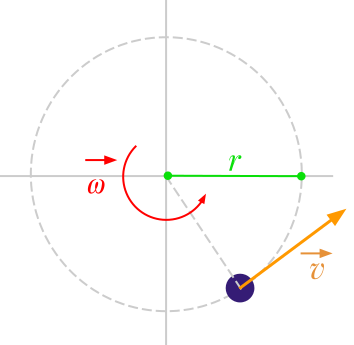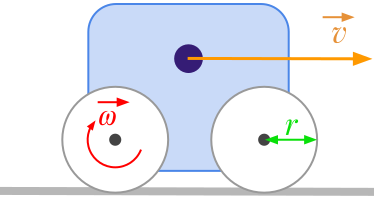Question #705

# How to convert rad/s to m/s?

Merged questionsThe formula to calculate the linear speed ﻿﻿ expressed in meters per second [﻿﻿] from the angular velocity ﻿﻿ expressed in radians per second [﻿﻿] is:

﻿﻿

where:

• ﻿﻿ is the linear velocity expressed in meters per second (﻿﻿)
• ﻿﻿ is the angular velocity expressed in radians per second (﻿﻿)
• ﻿﻿ is the radius expressed in meters (﻿﻿)For example, let's consider a vehicle with wheels having a radius of 10 centimetres. If the rotational velocity of the wheels is 20 rad/s, the linear speed of the vehicle is given by:

﻿﻿

15 events in history
Question by Alphonsio 05/14/2021 at 12:52:11 PM
How to convert an angular velocity to a linear velocity?
Question by Alphonsio 05/14/2021 at 12:51:38 PM
How to convert a angular speed to a linear velocity?
Question by Alphonsio 03/08/2021 at 06:32:38 PM
How to convert radians per second to meter per second?
Question by Alphonsio 03/01/2021 at 03:52:48 PM
How to convert rad/s to m/s?
Answer by Alphonsio 12/14/2020 at 07:14:45 PMThe formula to calculate the linear speed ﻿﻿ expressed in meters per second [﻿﻿] from the angular velocity ﻿﻿ expressed in radians per second [﻿﻿] is:

﻿﻿

where:

• ﻿﻿ is the linear velocity expressed in meters per second (﻿﻿)
• ﻿﻿ is the angular velocity expressed in radians per second (﻿﻿)
• ﻿﻿ is the radius expressed in meters (﻿﻿)For example, let's consider a vehicle with wheels having a radius of 10 centimetres. If the rotational velocity of the wheels is 20 rad/s, the linear speed of the vehicle is given by:

﻿﻿

Answer by Alphonsio 12/14/2020 at 07:14:36 PMThe formula to calculate the linear speed ﻿﻿ expressed in meters per second [﻿﻿] from the angular velocity ﻿﻿ expressed in radians per second [﻿﻿] is:

﻿﻿

where:

• ﻿﻿ is the linear velocity expressed in meters per second (﻿﻿)
• ﻿﻿ is the angular velocity expressed in radians per second (﻿﻿)
• ﻿﻿ is the radius expressed in meters (﻿﻿)For example, let's consider a vehicle with wheels having a radius of 10 centimetres. If the rotational velocity of the wheels is 20 rad/s, the linear speed of the vehicle is given by:

﻿﻿

Answer by Alphonsio 12/14/2020 at 07:13:35 PMThe formula to calculate the linear speed ﻿﻿ expressed in meters per second [﻿﻿] from the angular velocity ﻿﻿ expressed in radians per second [﻿﻿] is:

﻿﻿

where:

• ﻿﻿ is the linear velocity expressed in meters per second (﻿﻿)
• ﻿﻿ is the angular velocity expressed in radians per second (﻿﻿)
• ﻿﻿ is the radius expressed in meters (﻿﻿)For example, let's consider a vehicle with wheels having a radius of 10 centimetres. If the rotational velocity of the wheels is 20 rad/s, the linear speed of the vehicle is given by:

﻿﻿

Answer by Alphonsio 12/14/2020 at 07:13:03 PMThe formula to calculate the linear speed ﻿﻿ expressed in meters per second [﻿﻿] from the angular velocity ﻿﻿ expressed in radians per second [﻿﻿] is:

﻿﻿

where:

• ﻿﻿ is the linear velocity expressed in meters per second (﻿﻿)
• ﻿﻿ is the angular velocity expressed in radians per second (﻿﻿)
• ﻿﻿ is the radius expressed in meters (﻿﻿)For example, let's consider a if a vehicle with wheels having a radius of 10 centimetres. The rotational velocity of the wheels is 20 rad/s. The linear speed of the vehicle is given by:

﻿﻿

Answer by Alphonsio 12/12/2020 at 05:44:27 AM

The formula to calculate the linear speed ﻿﻿ expressed in meters per second [﻿﻿] from the angular velocity ﻿﻿ expressed in radians per second [﻿﻿] is:

﻿﻿

where:

• ﻿﻿ is the linear velocity expressed in meters per second (﻿﻿)
• ﻿﻿ is the angular velocity expressed in radians per second (﻿﻿)
• ﻿﻿ is the radius expressed in meters (﻿﻿)
Answer by Alphonsio 12/12/2020 at 05:43:53 AM

The formula to convert angular velocity ﻿﻿ expressed in radians per second [﻿﻿] to linear speed ﻿﻿ expressed in meters per second [﻿﻿] is given by:

﻿﻿

where:

• ﻿﻿ is the linear velocity expressed in meters per second (﻿﻿)
• ﻿﻿ is the angular velocity expressed in radians per second (﻿﻿)
• ﻿﻿ is the radius expressed in meters (﻿﻿)
Answer by Alphonsio 12/11/2020 at 07:45:54 PM

The formula to convert angular velocity expressed in radians per second [﻿﻿] to linear speed expressed in meters per second [﻿﻿] is given by:

﻿﻿

﻿﻿

where:

• ﻿﻿ is the linear velocity expressed in meters per second (﻿﻿)
• ﻿﻿ is the angular velocity expressed in radians per second (﻿﻿)
• ﻿﻿ is the radius expressed in meters (﻿﻿)
Answer by Alphonsio 12/11/2020 at 07:42:09 PM

The formula to convert angular velocity expressed in radians per second [﻿﻿] to linear speed expressed in meters per second [﻿﻿] is given by:

﻿﻿

﻿﻿

where:

• ﻿﻿ is the linear velocity expressed in meters per second (﻿﻿)
• ﻿﻿ is the angular velocity expressed in radians per second (﻿﻿)
• ﻿﻿ is the radius expressed in meters (﻿﻿)
Answer by Alphonsio 12/11/2020 at 07:42:03 PM

The formula to convert angular velocity expressed in radians per second [﻿﻿] to linear speed expressed in meters per second [﻿﻿] is given by:

﻿﻿

﻿﻿

where:

• ﻿﻿ is the linear velocity expressed in meters per second (﻿﻿)
• ﻿﻿ is the angular velocity expressed in radians per second (﻿﻿)
• ﻿﻿ is the radius expressed in meters (﻿﻿)

Answer by Alphonsio 12/11/2020 at 07:41:40 PM

The formula to convert angular velocity expressed in radians per second [﻿﻿] to linear speed expressed in meters per second [﻿﻿] is given by:

﻿﻿

﻿﻿

where:

• ﻿﻿ is the linear velocity expressed in meters per second (﻿﻿)
• ﻿﻿ is the angular velocity expressed in radians per second (﻿﻿)
• ﻿﻿ is the radius expressed in meters (﻿﻿)

Question by Alphonsio 12/11/2020 at 07:40:25 PM
How to convert radians per second to meters per second?

Icons proudly provided by Friconix.